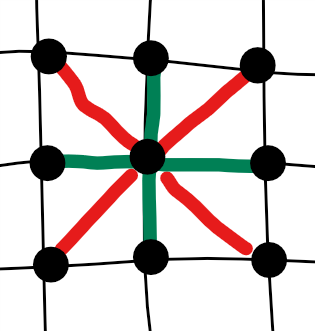2D Ising model with competing orders
$$S=K\sum_{\langle i,j\rangle} s_i s_j + V \sum_{\langle \langle i,j\rangle\rangle} s_i s_j$$ green bonds are nearest couplings $$\langle i,j\rangle$$; the red bonds are next nearest couplings $$\langle\langle i,j\rangle\rangle$$the system gets very rich phase diagram, for example, when

updating speed=100 Coupking $$K$$=0.5 Coupking $$V$$=0.5

### physics experiments you can do!

#### 1. explore the phases

when $$K=0$$, the system can be divided into two decoupled sub-systems.
$$V=-\infty$$ gives two anti-ferromagnetic system, according to their relative postition, we can have either vertial strip order or horizantal strip order.

#### 2. hysteresis effect

1. tune $$V$$ to the largest postive value
2. tune $$K$$ to zero.
3. set updating speed to maximum
4. wait some time, until domain wall disappear
5. now tune $$K$$ slowly from 0 to 0.2
6. and then tune $$K$$ slowly from 0.2 to -0.2
7. go back tune $$K$$ slowly from -0.2 to 0.2
8. you will see a hysteresis effect happen near $$K=\pm 0.14$$, for $$V=1$$

#### 3. neutron scattering

I will add FFT feature later, where you can see the scattering plot.